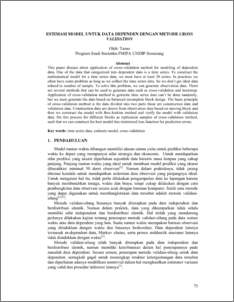# ESTIMASI MODEL UNTUK DATA DEPENDEN DENGAN METODE CROSS VALIDATION

Tarno, Tarno (2008) ESTIMASI MODEL UNTUK DATA DEPENDEN DENGAN METODE CROSS VALIDATION. Media Statistika, 1 (2). pp. 75-82. ISSN 1979-3693Preview
PDF - Published Version
118Kb

Official URL: http://stat.undip.ac.id

## Abstract

This paper discuss about application of cross-validation method for modeling of dependent data. One of the data that categorized into dependent data is a time series. To construct the mathematical model for a time series data, we must have at least 50 series. In practices we often have some problem as long as we collect the time series data. So we don’t get ideal data related to number of sample. To solve this problem, we can generate observation data. There are several methods that can be used to generate data such as cross-validation and bootstrap. Application of cross-validation method to generate time series data can’t be done randomly, but we must generate the data based on balanced incomplete block design. The basic principle of cross-validation method is the data divided into two parts those are construction data and validation data. Construction data are drawn from observation data based on moving block and then we construct the model with Box-Jenkins method and verify the model with validation data. Do this process for different blocks as replication samples of cross-validation method, such that we can construct the best model that minimized loss function for prediction errors. Key words: time series data, estimate model, cross-validation

Item Type: Article Q Science > Q Science (General) Faculty of Science and Mathematics > Department of Statistics 1392 INVALID USER 15 Oct 2009 15:46 15 Oct 2009 15:46

Repository Staff Only: item control page